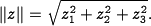# FAQ: What do the double vertical bars mean at the end of Section 4.1?

Jump to: navigation, search

(Contributed by Richard Murray, 28 August 2010)

In the text at the end of Section 4.1, the following equation occursThe expression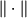represents the magnitude of the vector enclosed between the sets of double vertical bars. For example, if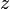is a vector of length three with elements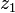,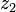,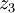, then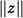is given by